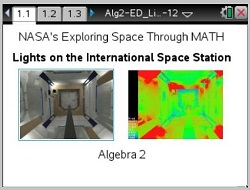Activities

••• Subject Area

• Math: Algebra II: Data Analysis

• Author9-12

• Device
•TI-Nspire™ CX/CX II
•TI-Nspire™ CX CAS/CX II CAS
• Software

TI-Nspire™
TI-Nspire™ CAS

3.2

Lights on the International Space StationActivity Overview

In this lesson, students will discover how various factors affect lighting by using different types of flashlights and battery strenghts.

Objectives

In this lesson, students will use the 5-E's Instructional Model - Engage, Explore, Explain, Extend, and Evaluate.

As a result, students will:

• analyze data from a simulation
• model data from a simulation using a graphical and algebraic approach
• apply the measure of illumination, lux=candela m2, to various situations

Vocabulary

• scatter plots
• modeling functions
• applying formulas
• inverse variation
• power functions